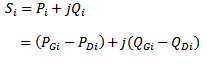Power flow study is a very important topic for power system engineering. Power flow studies are used to ensure that electrical power transfer from generation to consumers through the grid system is stable, reliable and economic. It helps us to make a proper design of power system for producing more energy. It also helps to do economical operation. It tells what is happening in the power system, how to perform under different operating condition. Power flow studies are often used to identify the need for additional generation, capacities support or the placement of capacitors, reactors to maintain system voltages within specified limits.

Power flow study

Power flow study is the steady state solution of the power system network.

The power flow equation

S = P12 + jQ12

=V1.I*12

So, power flow is two types –

1. Real power
2. Reactive power

Real power: The component of apparent power that represents true work; expressed in watts, it is equal to volt-amperes multiplied by the power factor.

It is denoted by P.

The unit of this power is watt (W).

Reactive power: Reactive power is a power that flows back and forth between the inductive windings of the generator and the inductive windings of motors, transformers etc., which are part of the electrical load. This power does no useful work in the electrical load nor does it present load to the engine.

It is denoted by Q.

The unit of this power is var (volt-ampere reactive) because re-active power does not transfer net energy to the load. Sometimes it is called “wattles” power.

Complex power: The complex power is the vector sum of real and re-active power.

It is denoted by S.

The unit of this power is VA (volt-ampere) because it is a product of rms (Root mean square) voltage and rms current.

Apparent power: The absolute vale of Complex power is called Apparent power.

It is denoted by ISI.

The unit of this is also VA.Now, net complex power is injected into the ith bus

Here,

• Pi = Real injected power
• Qi = Re-active injected power
• PGi = Local power generation Real power
• PDi = Real power demand at the bus
• QGi = Local power generation Re-active power
• QDi = Re-active power demand at the bus

Bus Classification

In power flow study it is assumed that loads are constant and generator terminal voltages are tightly regulated and therefore are constant. The main objective of the power flow study is to find the voltage magnitude and angle of each bus. Each bus in system has four variables: voltage magnitude, voltage angle, real power and reactive power. During the operation of the power system, each bus has two known variables and two unknowns. Actually there are three types of buses-

a) Load Bus (PQ Bus): This bus is not connected to a generator so that neither its voltage nor its real power can be controlled. On the other hand, the load is connected to this bus so active reactive power at this bus changes randomly. So, we have to assume the complex power value at this bus to solve load flow problem.

b) Generator Bus (PV Bus): This bus also called Voltage controlled bus because during the operation the voltage magnitude at this bus is kept constant. This magnitude of the voltage is kept constant by adjusting the current of a synchronous generator on the bus.

c) Slack Bus (Swing Bus): This bus is considered as the reference bus. It is connected to a high rating relative to the other generators. During the operation, the voltage of this bus is always specified and remains constant in magnitude (|V|=1.04p.u) and angle (δ=0). This bus is responsible for supplying the losses of the system during the operation.